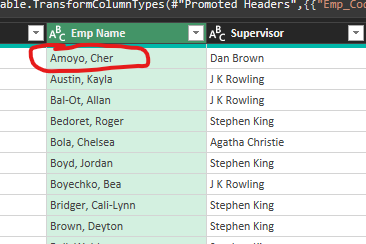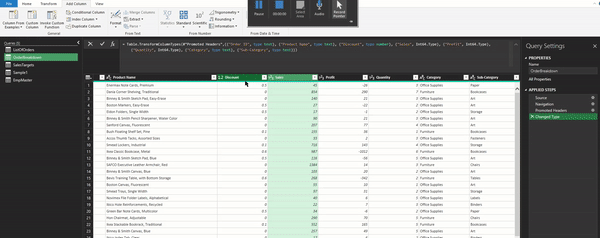Search

# Columns from Example: Add Columns in Power Query by giving examplesThe basic concept of machine learning is that we train the machine with a few samples and then use that acquired learning to give the outcome on the rest of the data set. We have seen this feature in Excel as Flash Fill.

Excel Quickbytes# 4: Flash Fill

The one challenge with the Flash Fill is that it does not consider any update in data automatically. If there is any change in the data, Flash Fill requires a re-run to give an updated result.

We can automate the process by using Column from Example in Power Query.

## How can Column from Examples be helpful?

• When we know the output required in the new column, but not sure which feature to select in Power Query.

• When we know which feature to use, but not sure about what to select to get the desired results

## Where to find Column from Examples?

We can find Column from Examples option under Add Column ribbon:It has two options:• From All Columns

• From Selection

We can use From All Columns when we know what output is required but are unsure about which columns to select to get the required output.

## Use Case# 1: Extracting information from a column.

Extracting year from data:Select the column > Add Column > Columns from Example > From Selection

Under Column > Type the expected result (in this case 2011), and hit CTRL enterIt adds a column with the year extracted from the parent column:Notice how Columns from Example adds the suitable M function for the action performed.Column from Examples is very intuitive, as it not adds a suitable formula, but also assigns a relatable header to the step.

## Use Case# 2: Creating our pattern.

In this example, we have the following name structure: [Last Name], [First Name]We can use Columns from Example to convert this structure to [First Name] [Last Name]In this example, it did not understand the output with one example; hence we repeat the first step one more time.

Furthermore, it applied the following formula for the step:## Use Case# 3: Conditional Column

We can also write a nested IF statement using Columns from Example. In this example, we want to categorize the sales amount in three categories: Less than 100, between 100 & 500, and More than 500.

Using Columns from Example, we demonstrate the output on a few sample data:It may not give the exact threshold we are looking for:Which we can edit:The benefit of Power Query is that the entire process is automated. Any update in the data table will reflect in the output.

## What are all transformations in the scope of Columns from Examples?

We can use it in the following transformations:

• Text Transformations

• Date Transformations

• Time Transformations

• Number Transformations

• Conditional Column

For more details, please refer to the article:

Add Column from Examples in Power BI Desktop

@imvivran